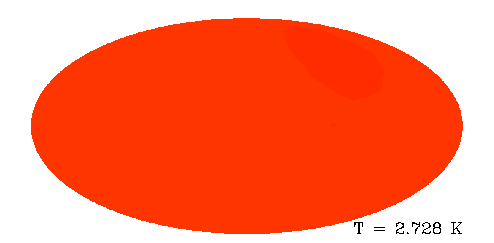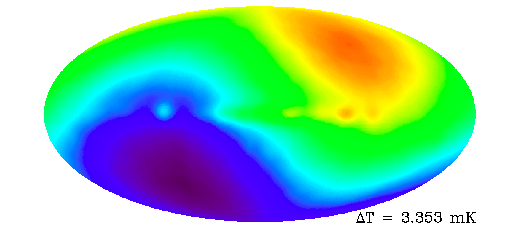Skip to main content
$$\require{cancel}$$

# 25: The Cosmic Microwave Background

### Discovery

As we have seen in our study of big bang nucleosynthesis, Alpher and Herman predicted the cosmic microwave background (CMB) in the 1940s. But it was decades until anyone mounted a serious campaign to look for this relic of the big bang. The delay seems astounding from our perspective, and yet nonetheless this is what happened. The prediction, of a relic of the big bang, that would not have been too difficult to detect, somehow did not garner much attention. The first effort to make measurements to detect the CMB was launched by a group at Princeton University in the early sixties. They had an extremely well-motivated set of observations in mind. They had the technical ability to build the instruments and make the measurements. They had the funding they needed. They just got started a little too late: in 1964 they were scooped by a pair of radio astronomers, a mere 30 miles to their east at Bell Labs, who had stumbled upon the cosmic relic accidentally. Life is not always fair.

Arno Penzias and Robert Wilson were the Bell Labs-employed radio astronomers, who, though they did not know it, were performing measurements of great historic importance. They had re-purposed a radio telescope, built for early experiments with satellite communication, to do some astronomy, such as measuring radio emission from a supernova remnant. As part of a process of characterizing their instrument and its noise properties, they made measurements away from any such sources. They were unable to reconcile the output from their radiometer, when the telescope was aimed at blank sky, with their estimates of the amount of noise there should be in their measurements. No matter where they pointed their telescope, the radiometer output exceeded what they expected from noise, consistent with a uniform background of microwaves.

The two groups became aware of each other's efforts (via some intermediaries), which led to them all realizing that the CMB had been detected. They agreed to write a pair of papers. The first was authored by Penzias and Wilson and titled "A Measurement of Excess Antenna Temperature at 4080 Mc/s." The second was written by the Princeton group and provided the cosmological interpretation of their measurement, simply titled "Cosmic Black-body Radiation." Penzias and Wilson received the 1978 Nobel Prize in Physics for their discovery.

## Spectrum of the CMB

#### Theoretical Prediction

According to our standard thermal history, reactions that create and destroy photons were fast enough at $$z \ge 10^7$$ to drive the CMB photons into a black body distribution; i.e., a thermal distribution with zero chemical potential (for massless bosons). In terms of the phase space distribution function, $$f$$ we have

$f = \frac{1}{\exp(pc/k_BT) -1}.$

So that's true for $$z \ge 10^7$$. What about today? We need to work that out in order to compare with observations we can do today. At $$z \le 10^7$$ the number changing reactions are slow so we are not guaranteed that the photon chemical potential remains zero. Let's work out what happens under the assumption that no new energy is injected into the photon distribution (by an unstable particle that has decay byproducts that include photons, for example). Let's do so by tracking what happens to photons in a small region of momentum space initially centered on momentum $$\vec p_i$$ with volume $$V_{p,i}$$, and in some comoving volume, with initial physical volume $$V_{x,i}$$. We've taken the phase space volume to be small enough that $$f$$ does not vary by much across it. So we don't need to integrate to find the total number of particles, we can just multiply:

$N_i = f(p_i;T_i) V_{x,i} V_{p,i}.$

Let's, for now, ignore scattering interactions that the photons have and treat them as if they are not receiving any kinetic energy from scattering. In this case their momenta are just redshifting with the expansion so that at some later point in evolution, when the scale factor has gone from $$a_i$$ to $$a_f$$, we have $$p_f = p_i a_i/a_f$$. Also due to the redshifting of momentum, the momentum-space volume that contains these photons shrinks by a factor of $$(a_i/a_f)^3$$, and the physical volume of the comoving volume we are tracking grows by $$(a_f/a_i)^3$$. So we have

\begin{align} N_f &= f(p_f;T_i) V_{x,f}V_{p,f} \\[4pt] &= f(p_f;T_i) \left(\dfrac{a_f}{a_i}\right)^3V_{x,i}\left(\dfrac{a_i}{a_f}\right)^3V_{p,i} \\[4pt] &= f(p_f;T_i) V_{x,i}V_{p,i}. \end{align}

Box $$\PageIndex{1}$$

Exercise 25.1.1: Draw the Cartesian axes of a 2-dimensional momentum space ($$p_x,p_y$$) and also of a 2-dimensional configuration space ($$x,y$$). Draw a box centered on the origin of the configuration space and one around some region of the momentum space, representing boxes bounding the particles we are going to track from one time to another. Take this first drawing as for the initial time, and make a subsequent pair of graphs for the later time, with the sizes and locations of these boxes evolving appropriately, following the above discussion. Take the configuration space coordinates to represent physical distances of particles from the origin; i.e., these are not comoving coordinates.

How is $$N_f$$ related to $$N_i$$? By design we've tried to keep these two equal. We've shifted the region of momentum space to track the photons as they redshift. So none of them have moved out of our momentum-space region due to how the momentum has changed. They are moving through space in different directions, so some will leave our comoving spatial volume. But, due to homogeneity and isotropy, the same number will flow in as flow out. The net result is that $$N_f = N_i$$. From this we can conclude that

\begin{align} f(p_f;T_i, t_f) = f(p_i;T_i,t_i) &=\frac{1}{\exp(p_ic/k_BT_i)-1} \\[4pt] &= \frac{1}{\exp(p_f (a_f/a_i)c/k_BT_i)-1 }= \frac{1}{\exp(p_f c/(k_BT_i a_i/a_f))-1} \\[4pt] &= \frac{1}{\exp(p_fc/k_BT_f)-1} \end{align}

with $$T_f = T_i \dfrac{a_i}{a_f}$$.

This is a remarkable result. Even though we ignored all interactions, the things that maintain equilibrium, we find out that such evolution results in a phase space distribution function that is the same as one would get for thermal equilibrium with zero chemical potential, although at a reduced temperature. The expansion of the universe cools the gas of photons while maintaining a chemical-potential-free thermal distribution.

We have ignored any energy that might be imparted to the photons by the electrons. But any such energy is very small since, in a given region of space, the total kinetic energy in the electrons is much less than the total kinetic energy in the photons, mainly because of how the photons greatly outnumber the electrons. The bottom line is that we expect, to a good approximation, the photons today to have a phase space distribution function for massless bosons in thermal equilibrium with zero chemical potential. Given that they have two degrees of freedom, this is a Planck distribution.

#### The Spectrum Observed

NASA's COsmic Background Explorer (COBE) satellite was launched in 1989 with three scientific instruments on board, two of which would lead to Nobel prize-worthy discoveries. One of these two was the Far Infrared Absolute Spectrometer, or FIRAS. The precision and accuracy of the FIRAS measurements were extraordinary. The figure below shows the spectrum of the CMB as determined from FIRAS data. The measurements are so precise that the width of the error bars is smaller than the thickness of the theoretical black body spectrum curve. You can read the original paper describing the FIRAS measurements and determination of this spectrum here: https://iopscience.iop.org/article/10.1086/178173/pdf.## Mapping the millimeter-wave sky

The radiation that we measure in the sky today comes from a thin spherical shell around us called "the surface of last scattering." This is the region of space (currently around 46 billion light years from the Earth) that is just the right distance from us, that the light traveling since decoupling is just getting here now.

## The CMB Monopole

The CMB is so uniform that a full-sky map of the CMB intensity, if the average (or "monopole") has not been subtracted, looks completely uniform.  The full-sky map below was made with data from the second Nobel-prize-winning instrument on the COBE satellite, the Differential Microwave Radiometer (DMR). The high degree of uniformity of this map reflects the high degree of uniformity of the universe at the epoch of decoupling.Figure $$\PageIndex{2}$$: The CMB as mapped by the COBE satellite. The single color shows the overall isotropy of the CMB.

## The CMB Dipole

When we make measurements of remnants of the early universe, there is something we must remember: Earth is not at rest. The Earth moves around the Sun, which orbits the center of the Milky Way. The Milky Way galaxy itself is falling towards Andromeda and the Local Group of galaxies is falling toward the Virgo Cluster. It is no surprise then that we are moving with respect to the rest frame of the cosmic microwave background. Our relative motion induces a dipole pattern on the sky, as can be seen in the map below which has had the monopole subtracted from it. We see a dipole pattern, as well as contamination by some emission from our own galaxy clustered near a horizontal line that goes through the center of the map. Radiation in the Earth's direction of motion appears blueshifted and hence hotter, while radiation on the opposite side of the sky is redshifted and colder.Figure $$\PageIndex{3}$$: The CMB as mapped by the COBE satellite, after monopole subtraction. The dipole component becomes clearly visible .

Box $$\PageIndex{2}$$

Exercise 25.2.1: Provide a 6 to 10-sentence summary of this chapter.

• Was this article helpful?